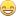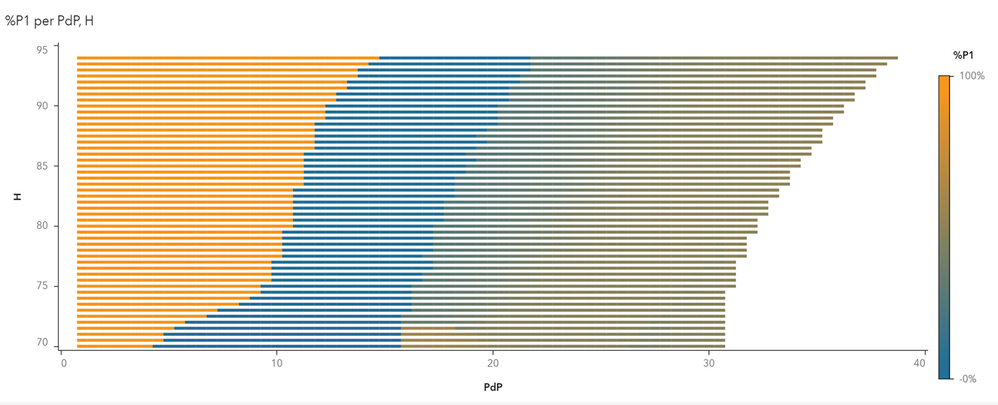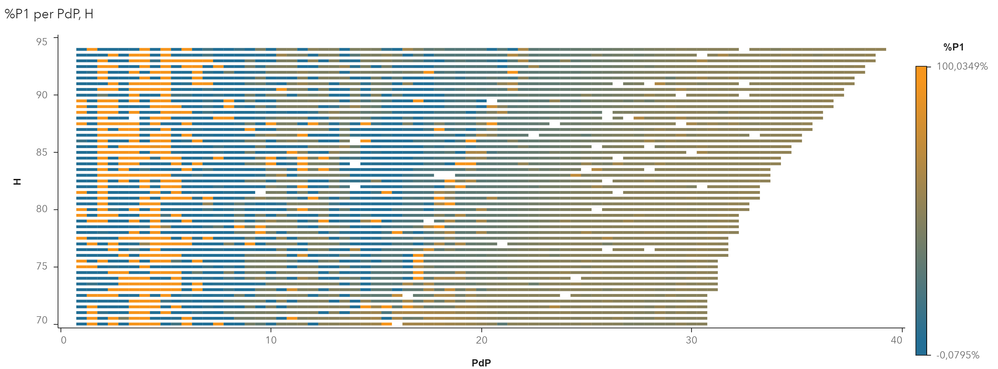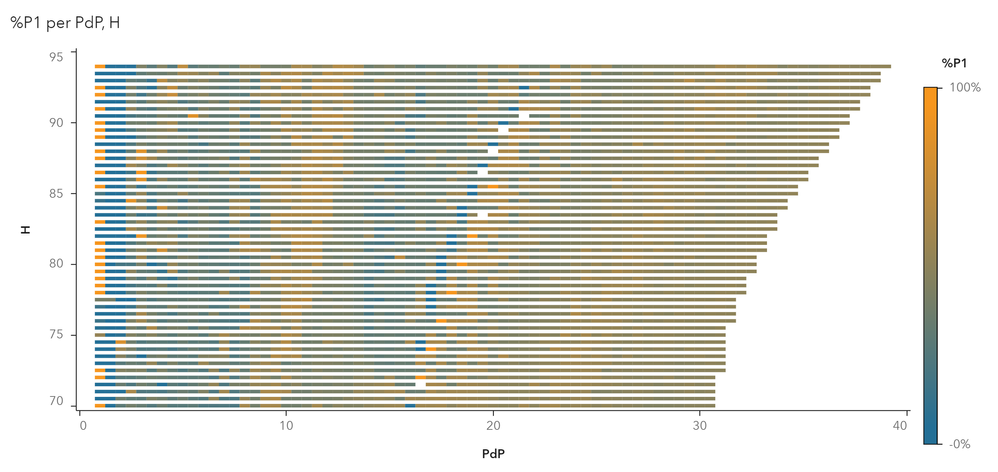## Constraint with disjoint intervals

I need to set a constraint like this: x in [1,2] or x = 0

I tried with

`constraint c1: 1 <= x <=2 or x = 0`

but it doesn't work. What is the right syntax?

Thanks

Regards

12 REPLIES 12

## Re: Constraint with disjoint intervals

``````proc optmodel;
var X >= 0 <= 2;
var Y binary;

/* if Y = 0 then X <= 0, else X <= X.ub (redundant) */
con C1: X <= X.ub * Y;

/* if Y = 1 then X >= 1, else X >= 0 (redundant) */
con C2: X >= Y;
quit;``````

## Re: Constraint with disjoint intervals

Thanks! Unfortunately my problem is non linear, so the solver is nlp.

And using binary variables, it says:

`ERROR: The NLP solver does not allow integer variables.`

## Re: Constraint with disjoint intervals

What does the rest of your model look like?

## Re: Constraint with disjoint intervals

Here is the whole model:

``````proc fcmp outlib=work.funcs.f;
function f1(Q, H);
return (
(7.18895803108255)+(Q)*(0.90898166129053)+(H)*(-0.52518338771685)+(Q**2)*(-0.07243939757267)+(Q)*(H)*(-0.0101157174044)+(H**2)*(0.01140027697845)+(Q**3)*(0.00271247731414)+(Q**2)*(H)*(0.00078575662965)+(Q)*(H**2)*(-8.0331130545237E-7)+(H**3)*(-0.00010011945215)+(Q**4)*(-0.00005707623607)+(Q**3)*(H)*(-0.0000145464761)+(Q**2)*(H**2)*(-4.0740624504878E-6)+(Q)*(H**3)*(5.6715539778245E-7)+(H**4)*(3.0399728809992E-7)+(Q**5)*(6.2706019750555E-7)+(Q**4)*(H)*(-4.9275589970883E-8)+(Q**3)*(H**2)*(1.1402170133176E-7)+(Q**2)*(H**3)*(-1.1450221400824E-8)
);
endsub;

function f2(Q, H);
return (
(9.56532591440209)+(Q)*(1.56477959347719)+(H)*(-0.58834848839521)+(Q**2)*(-0.14923333670627)+(Q)*(H)*(-0.02042582402532)+(H**2)*(0.01042030607175)+(Q**3)*(0.00551139580932)+(Q**2)*(H)*(0.00200710134061)+(Q)*(H**2)*(0.00006229314138)+(H**3)*(-0.00006493899805)+(Q**4)*(-0.00009774971067)+(Q**3)*(H)*(-0.00004756024059)+(Q**2)*(H**2)*(-0.00001062838926)+(Q)*(H**3)*(3.7950591182214E-7)+(Q**5)*(8.5938390272548E-7)+(Q**4)*(H)*(1.7658335207072E-7)+(Q**3)*(H**2)*(2.2050828738805E-7)+(H**5)*(8.7276517928309E-10)
);
endsub;

function g1(Q1,Q2); return ( 0.003134  * ((Q1+Q2)**2) + 0.003089 * (Q1**2) ); endsub;
function g2(Q1,Q2); return ( 0.003134  * ((Q1+Q2)**2) + 0.003089 * (Q2**2) ); endsub;
run;

%let Q1max = 30.4;
%let Q2max = 29.5;
%let P1min = 2.5;
%let P2min = 3.3;
%let P1max = 21.7;
%let P2max = 21.6;
%let PSETL = 1;
%let PSETR = 43;
%let HSETL = 70;
%let HSETR = 94;
%let STEP  = 0.5;

options cmplib=work.funcs;

number P;
number H;

var Q1 >= 0 <= &Q1max;
var Q2 >= 0 <= &Q2max;

impvar HL1 = H - g1(Q1,Q2);
impvar HL2 = H - g2(Q1,Q2);

impvar P1 = Q1 * HL1 * f1(Q1, HL1) * 9.81 / 1000;
impvar P2 = Q2 * HL2 * f2(Q2, HL2) * 9.81 / 1000;

minimize Q = Q1 + Q2;

constraint P = P1 + P2;

constraint 0 <= P1 <= &P1max; /* (*) */
constraint 0 <= P2 <= &P2max; /* (*) */

set PSET = &PSETL .. &PSETR by &STEP;
set HSET = &HSETL .. &HSETR by &STEP;

num Qopt {PSET, HSET};
num Q1opt {PSET, HSET};
num Q2opt {PSET, HSET};
num P1opt {PSET, HSET};
num P2opt {PSET, HSET};
str solstatus {PSET, HSET};
num opterror {PSET, HSET};
do P = PSET;
do H = HSET;
solve with nlp / maxiter=10000 multistart=(maxstarts=10) seed=54321;
put P= H= HL1= HL2= P1= P2= Q1= Q2= _solution_status_=;
Qopt[P,H] = Q.sol;
Q1opt[P,H] = Q1.sol;
Q2opt[P,H] = Q2.sol;
P1opt[P,H] = P1;
P2opt[P,H] = P2;
solstatus[P,H] = _solution_status_;
opterror[P,H] = _OROPTMODEL_NUM_['OPTIMALITY_ERROR'];
end;
end;
create data Qdata from [P H] P1opt P2opt Q1opt Q2opt Qopt solstatus opterror;
quit;

``````

Look at the rows marked with (*)

``````constraint 0 <= P1 <= &P1max; /* (*) */
constraint 0 <= P2 <= &P2max; /* (*) */``````

As you can see, P1 and P2 have 0 as lower bound, and it worked very well. Now I'm asked to restrict P1 and P2 interval from [0,PMAX] to [PMIN, PMAX], but zero must remain allowed, so the constraints should become

```(P1 = 0) OR (&P1min <= P1 <= &P1max)
(P2 = 0) OR (&P2min <= P2 <= &P2max)```

Hence this question. I hope I made myself clear now!

Thanks again,

Regards

## Re: Constraint with disjoint intervals

I have suggestions for two alternative approaches.

One approach is to use the LSO solver:

``````   var Y1 binary;
con P1 <= &P1max * Y1;
con P1 >= Y1;
var Y2 binary;
con P2 <= &P2max * Y2;
con P2 >= Y2;   /* and then replace the SOLVE statement as follows */    solve with lso;``````

The second approach, based on the fact that you have only two disjunctions is to solve four problems and keep the best solution:

1. Fix P1 = 0 and P2 = 0.
2. Fix P1 = 0 and impose range constraint &P2min <= P2 <= &P2max.
3. Fix P2 = 0 and impose range constraint &P1min <= P1 <= &P1max.
4. Impose two range constraints &P1min <= P1 <= &P1max and  &P2min <= P2 <= &P2max.

By the way, the following additional approaches are valid syntax but unlikely to work well:

``````   constraint (P1 = 0 OR &P1min <= P1 <= &P1max) >= 1;
constraint (P2 = 0 OR &P2min <= P2 <= &P2max) >= 1;
``````
``````   constraint (P1 = 0) + (&P1min <= P1 <= &P1max) >= 1;
constraint (P2 = 0) + (&P2min <= P2 <= &P2max) >= 1;
``````

## Re: Constraint with disjoint intervals

Thanks, we are getting closerAbout the first approach with lso. I tried to run the model without changing the constraints (so my original problem) to see what are the results comparing nlp with lso.

Let me explain the result: the variables the model finds are Q1 and Q2, for every P and H in a grid (as you can see in the code); then I calculate P1 and P2, and since P1+P2=P I calculate the ratio P1/P to get one number as result; in this way, when the ratio is 100% it means that P1=P and P2=0, when it is 0% it means that P1=0 and P2=P, otherwise 0 < P1,P2 < P.

In the following graph I display in the P,H grid, this ratio with color: yellow (ratio=100%) blue (ratio=0%) brownish (0 < ratio < 100).

Here's the solution with nlp:Here's the solution with lso:The solution with nlp makes a lot of sense (from the physical point of view of the thing I'm optimizing): when P is low, P1=P and P2=0; when P is medium, P1=0 and P2=P; when P is high, a combination of P1 and P2 is used. And you can see from the picture a sense of "continuity" in the grid. I know it may sound obscure but trust me this result is good!

Now the solution with lso seems a lot more noisy: the sense of "continuity" seems not to apply, and also the P low/medium/high is unclear. So before modify the constraint to fit the new problem of this thread, I'd like to get a more stable solution with lso, as "good" as the nlp one. Do you know if the lso can be configured to be more accurate? I read the doc and I saw a lot of parameters, I'm not sure about what to do to improve the results.

As for the second approach: yes I taught about this, and I believe it works fine. However for now I wrote the model with two "units" (you can see the reference to indexes "1" and "2" all over the code), but I'm sure I will be asked later to generalize it to work with N "units". So with N "units", I should have to solve 2^N-1 problems with all the combinations of P_i (zero or non zero) and then compare them, and it sounds hard...

As for the thirds approach, I haven't tried yet, but I think it will not be accurate at all since in this way the error on constraints won't have a metric to see how wrong it is, it would only get a flag "correct" "incorrect".

Thanks again,

Regards

## Re: Constraint with disjoint intervals

Could you please try the experiment with these options:

solve with lso / absfconv=1e-10 nabsfconv=100 maxgen=500 popsize=500 feastol=1e-7;

Increasing the popsize often improves the solution quality.

## Re: Constraint with disjoint intervals

Unfortunately the solution got worse...

Here's the result, and most of the points have status MAXGEN instead of ABSFCON.## Re: Constraint with disjoint intervals

That is unfortunate.  It may be LSO is not a good fit.

I did try the first problem to verify LSO gave comparable results, but did not have time to run through the entire list.  If you had an instance in the loop where the objective Q.sol is much worse, that would help to diagnose.

Looking closer, I didn't realize the constraints were nonlinear.  The feastol is likely too tight as LSO is a DFO algorithm.

If you have time/interest, could you rerun but replace the solve command with:

solve with lso / absfconv=1e-6 nabsfconv=100 maxgen=100 popsize=500 feastol=1e-3;
solve with nlp;

The second nlp solve is to increase the feasibility of the solution found.  When moving to binary we can can add a relaxint option that may work analogously for the mixed integer case.

Thanks.

## Re: Constraint with disjoint intervals

Yes the constraints are nonlinear, but they are "nice" (in the sense that they are almost always polynomials and in general continuous and derivable, that is why I expect this sense of continuity of the solutions over the grid).

I picked up these two instances:

1)

P=6, H=85.

With nlp: Q1=9.58, Q2=0.00, Q=9.58 (status=OPTIMAL).

With lso: Q1=5.30, Q2=7.60, Q=12.90 (status=MAXGEN).

2)

P=10, H=91.5.

With nlp: Q1=13.63, Q2=0.00, Q=13.63 (status=OPTIMAL).

With lso: Q1=7.26, Q2=9.52, Q=16.78 (status=ABSFCON).

I'll try to rerun it with the double solve command.

In the mean time I think I'll start to implement approach no.2, let's see if it's reliable.

Thanks again for all you suggestions!

Regards

## Re: Constraint with disjoint intervals

Thanks a lot for the two examples!  I was able to get similar solutions (using sledgehammer sized population:) if I used these two solve commands:

solve with lso / absfconv=1e-6 nabsfconv=10 maxgen=10 popsize=10000 feastol=1e-2;
solve with nlp /algorithm=as;

But this could be a game of wack-a-mole.  Also it is much slower ... so likely a dead-end unless no 2 does not help.

Thanks a lot for the examples.  For better or worse, LSO treats all problems as black-boxes and avoids assumptions about derivatives existing.  So it may continue to optimize when other solvers get stuck--the price is when derivatives do exist it will still tick-tack to the solution.  Thus in general, if everything insight is smooth, the nlp solvers are more appropriate.  But adding binary variables will of course induce some (albeit structured) non-smoothness ...

## Re: Constraint with disjoint intervals

This is something we could do for you in the next release.  But for now would need to be done manually:

One potential heuristic to overcome this hurdle if N "units" is greater than 2 is to create a binary LHS sampling (over which variables should be fixed at 0 and which should be free) that predetermines which variables will get fixed for a set of B "tests".  There is example code here: